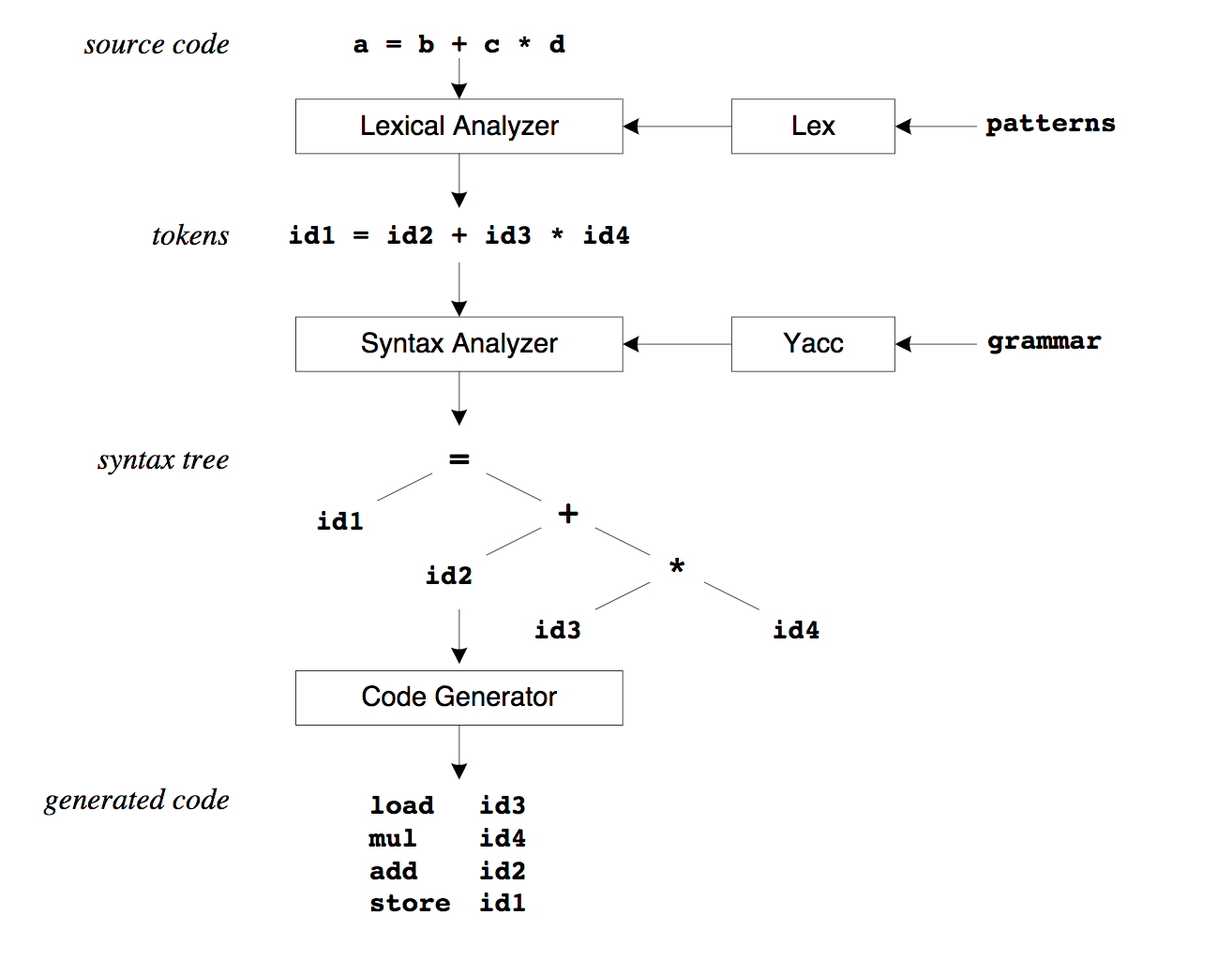# Ruby Ruby 字符串解析引擎 Racc 和 Rex 分析

dengqinghua · 2018年05月29日 · 3636 次阅读

## Racc

• Rex 和 Racc 的关系
• 如何使用 Racc 进行自定义

## TL;DR

Ruby 中我们使用 sql-parser

``````require 'sql-parser'
parser = SQLParser::Parser.new

# Build the AST from a SQL statement
ast = parser.scan_str('SELECT * FROM users WHERE id = 1')

# Find which columns where selected in the FROM clause
ast.select_list.to_sql
#=> "*"

# Output the table expression as SQL
ast.table_expression.to_sql
#=> "FROM users WHERE id = 1"

# Drill down into the WHERE clause, to examine every piece
ast.table_expression.where_clause.to_sql
#=> "WHERE id = 1"
ast.table_expression.where_clause.search_condition.to_sql
#=> "id = 1"
ast.table_expression.where_clause.search_condition.left.to_sql
#=> "id"
``````

1. patterns, 用 `parser.rex` 来配置, 如 SELECT, ORDER 等词汇
2. grammar, 用 `parser.racc` 来配置, 定义了语法, 即 SELECT, ORDER 的组合方式

``````SELECT * FROM orders WHERE id = 1
``````

``````SELECT
*
FROM
WHERE
``````

## Rex 和 Racc 的来源

### Yacc 和 Lexical

Racc 和 Rex 分别来源于单词 Yacc`Lexical Analyser`

1. 有哪些词汇 (patterns)?
2. 有哪些语法 (grammar)?## Rex

### 示例

``````macro
DIGIT_MACRO     \d+

rule
{DIGIT_MACRO} { [:DIGIT, text.to_i] }
``````

``````输入 1024, 解析为词汇 [:DIGIT, 1024]
``````

NOTE: 参考: a-tester-learns-rex-and-racc-part-1

## Racc

### 示例

``````rule
expression
:
DIGIT
| DIGIT ADD DIGIT { return val + val }
| DIGIT SUBSTRACT DIGIT { return val - val }
| DIGIT MULTIPLY DIGIT { return val * val }
| DIGIT DIVIDE DIGIT { return val / val }
end
``````

1. expression 仅仅是一个名词, 可以起名为 abc, bcd 都行, 他可以被复用, 即规则可以嵌套使用. ":"后面是代表各种不同的情况, | 代表 或
2. DIGIT ADD SUBSTRACT 等, 均为 calculator.rex 中申明的词汇 (patterns)
3. val 是指匹配成功之后, 对应的值

如字符串 `2 + 2`, 匹配到了 `DIGIT ADD DIGIT` 这部分, 则

``````val = 2
val = "+"
val = 2
``````
4. {} 表示的是 ruby 代码, 即如何处理该语法.

## 编译

``````rex calculator.rex -o calculator.rex.rb
racc calculator.y -o calculator.racc.rb
``````

NOTE: 在这之前需要安装 racc 这个 gem. 可以通过 `gem install racc` 进行安装

NOTE: 希望之后可以添加 Rspec 测试, 这样更加直观和规范

``````pry -r ./calculator.racc.rb
``````

``````Calculator.new.parse("2 + 2") #=> 输出 4
Calculator.new.parse("2 - 2") #=> 输出 0
Calculator.new.parse("2 * 3") #=> 输出 6
Calculator.new.parse("2 / 1") #=> 输出 2
Calculator.new.parse("2 | 1") #=> 抛出异常, 因为 | 无法解析: parse error on value 2 (DIGIT)
``````